# Exercise E6.3 Lines and Angles NCERT Solutions Class 9

Go back to  'Lines and Angles'

## Chapter 6 Ex.6.3 Question 1

In Fig. below, sides $$QP$$ and $$RQ$$ of $$∆ PQR$$ are produced to points $$S$$ and $$T$$ respectively. If $$\angle SPR = 135^\circ$$ and $$\angle PQT = 110^\circ$$ , find $$\angle PRQ$$.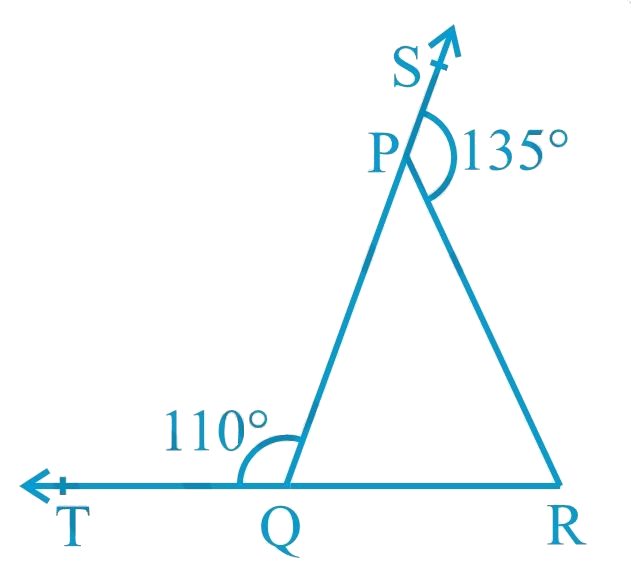### Solution

What is known?

$$\angle SPR = 135^\circ$$ and $$\angle PQT = 110^\circ$$

What is unknown?

$$\angle PRQ$$

Reasoning:

As we know the linear pair axioms:

If Non-common arms of two adjacent angles form a line, then these angles are called linear pair of angles and the sum of the linear pair is $$180^\circ$$.

If the sum of two adjacent angles is $$180^\circ$$ then the two non-common arms of the angles form a line.

Angle sum property of a triangle:

Sum of the interior angles of a triangle is $$360^\circ$$.

Steps:

Given,

$$\angle SPR = 135^\circ$$ and $$\angle PQT = 110^\circ$$

\begin{align}\angle SPR + \angle QPR &= 180^\circ \\ {\text{By linear pair axiom}} \\\\135^\circ + \angle QPR &= 180^\circ \\\angle QPR &=180^\circ\! -135^\circ \\\angle QPR &= 45^\circ.... {\rm{ }}\left( {\rm{i}} \right)\end{align}

\begin{align}\angle PQT + \angle PQR &= 180^\circ \\\begin{array}{I}(\text{By linear pair axiom}) \end{array}\\\\110^\circ + \angle PQR &= 180^\circ \\\angle PQR &= 180^\circ - 110^\circ \\\angle PQR &= 70^\circ..\left( {{\rm{ii}}} \right)\end{align}

Now,

\begin{align}\angle PQR + \angle QPR + \angle PRQ &= 180^\circ\\ (\text{Angle sum } \text{property of a}&\text{ triangle}) \\\\70^\circ + {\rm{ }}45^\circ + \angle PRQ &= 180^\circ\\{\rm{}}\left[ {{\rm{from }}\left( {\rm{i}} \right){\rm{ and }}\left( {{\rm{ii}}} \right)} \right]\\\\\angle PRQ &\!=180^\circ\!\! -\!115^\circ \\\angle PRQ& = 65^\circ \end{align}

## Chapter 6 Ex.6.3 Question 2

In Fig. below, $$\angle X = 62^\circ ,{\rm{ }}\angle XYZ = 54^\circ$$. If $$YO$$ and $$ZO$$ are the bisectors of $$\angle XYZ$$ and $$\angle XZY$$ respectively of $$\Delta XYZ,$$ find $$\angle OZY$$ and $$\angle YOZ$$ .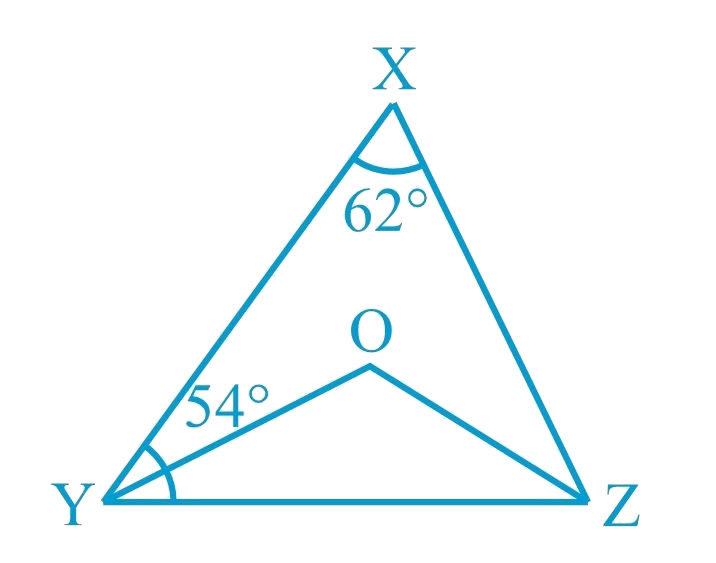### Solution

What is known?

$$\angle X = 62^\circ ,{\rm{ }}\angle XYZ = 54^\circ$$ and $$YO$$ and $$ZO$$ are the bisectors of $$\angle XYZ$$ and $$\angle XZY$$ respectively.

What is unknown?

$$\angle OZY$$ and $$\angle YOZ$$

Reasoning:

As we know the angle sum property of a triangle:

Sum of the interior angles of a triangle is $$360^\circ$$.

Steps:

Given, in $$\Delta XYZ$$,

\begin{align}\angle X &= 62^\circ \\\angle XYZ &= 54^\circ \end{align}

\begin{align}\angle X + \angle XYZ + \angle Z &= 180^\circ\\ ( \text{Angle sum property of }&\text{a triangle}\rm{.} )\\\\62^\circ + {\rm{ 5}}4^\circ + \angle Z &= 180^\circ \\\angle Z &=180^\circ-116^\circ \\\angle Z &= 64^\circ \end{align}

Now, $$OZ$$ is angle bisector of $$\angle XZY$$

\begin{align} \angle OZY = \frac{1}{2}\angle XZY &= \frac{1}{2} \times 64^\circ \\&= 32^\circ {\rm{ }}\left( {\rm{i}} \right)\end{align}

Similarly, $$OY$$ is angle bisector of $$\angle XYZ$$

\begin{align} \angle OYZ = \frac{1}{2}\angle XYZ &= \frac{1}{2} \times 54^\circ \\ &= 27^\circ \left( {{\rm{ii}}} \right)\end{align}

Now, in $$\Delta OYZ$$

\begin{align}\angle OYZ \!+\! \angle OZY \!+\! \angle YOZ &= 180^\circ \\( \text{Angle sum property of a }&\text{triangle}\rm{.} )\\\\27^\circ +32^\circ + \angle YOZ & = 180^\circ \\\left[ {{\textrm{from }}\left( {\rm{i}} \right){\rm{ and }}\left( {{\rm{ii}}} \right)} \right]\\\\\angle YOZ &\!= 180^\circ \!- 59^\circ \\\angle YOZ &= 121^\circ \end{align}

Hence, $$\angle OZY = 32^\circ$$ and $$\angle YOZ = 121^\circ$$

## Chapter 6 Ex.6.3 Question 3

In Fig. below, if $$AB || DE$$, $$\angle BAC = 35^\circ$$ and $$\angle CDE = 53^\circ$$, find $$\angle DCE$$.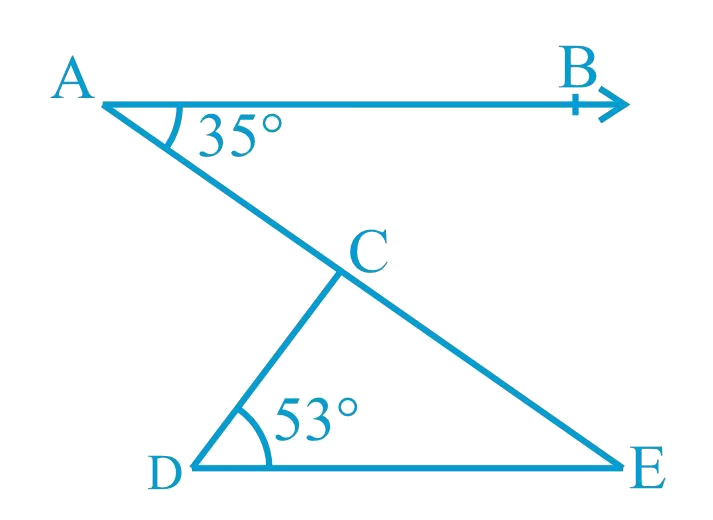### Solution

What is known?

$$AB || DE$$, $$\angle BAC = 35^\circ$$ and $$\angle CDE = 53^\circ$$

What is unknown?

$$\angle DCE$$

Reasoning:

As we know when two parallel lines are cut by a transversal, alternate interior angles formed are equal.

Angle sum property of a triangle:

Sum of the interior angles of a triangle is $$360^\circ$$.

Steps:

Given,

$$AB || DE$$ , $$\angle BAC = 35^\circ$$ and $$\angle CDE = 53^\circ$$

\begin{align}\angle DEC &= \!\angle BAC\\( \text{Alternate }&\text{interior angles})\\\\\angle DEC &= 35^\circ \end{align}

Now, in $$\Delta CDE$$

\begin{align}\angle CDE + \angle DEC + \angle DCE &= 180^\circ\quad \\( \text{Angle sum property of a}&\text{ triangle.})\\\\53^\circ + 35^\circ + \angle DCE &= 180^\circ \\\angle DCE &\!= 180^\circ \! - 88^\circ \\\angle DCE &= 92^\circ\end{align}

## Chapter 6 Ex.6.3 Question 4

In fig. below, if lines $$PQ$$ and $$RS$$ intersect at point $$T$$, such that $$\angle PRT = 40^\circ$$ ,$$\angle RPT = 95^\circ$$ and $$\angle TSQ = 75^\circ$$ , find $$\angle SQT$$ .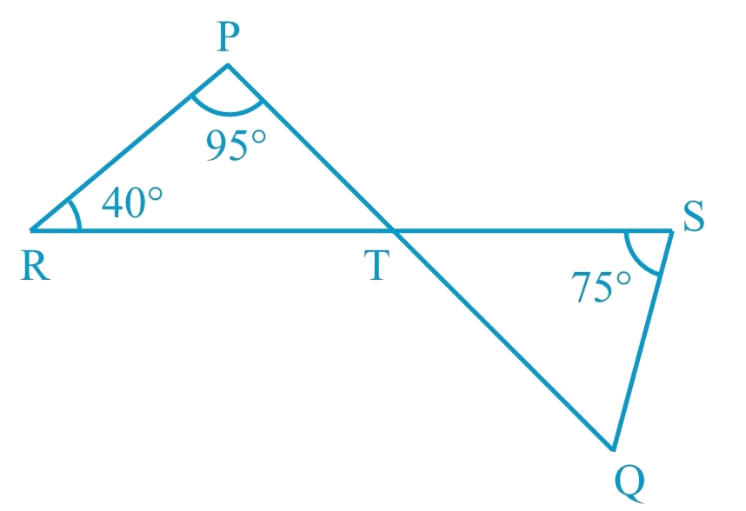### Solution

What is known?

$$\angle PRT = 40^\circ$$, $$\angle RPT = 95^\circ$$ and $$\angle TSQ = 75^\circ$$

What is unknown?

$$\angle SQT$$

Reasoning:

As we know when two line intersect each other at a point then there are two pairs of vertically opposite angles formed are equal.

Angle sum property of a triangle:

Sum of the interior angles of a triangle is $$360^\circ$$.

Steps:

Given,

$$\angle PRT = 40^\circ$$, $$\angle RPT = 95^\circ$$ and $$\angle TSQ = 75^\circ$$

In $$\Delta PRT$$

\begin{align}\angle PTR + \angle PRT + \angle RPT &= 180^\circ {\rm{ }}\\( \text{Angle sum property of a}&\text { triangle}\rm{.})\\\\\angle PTR + 40^\circ + 95^\circ &= 180^\circ \\\angle PTR &= 180^\circ - 135^\circ\\\angle{PTR} &= 45^\circ {\rm{ }}\end{align}

Now,

\begin{align}\angle QTS& = \angle PTR\quad\\( \text{Vertically }&\text {opposite angles})\\\\\angle QTS &= 45^\circ\quad \left( {\rm{i}} \right)\end{align}

In $$\Delta TSQ$$

\begin{align}\angle QTS + \angle TSQ + \angle SQT &= 180^\circ\\( \text{Angle sum property of a }&\text {triangle}.)\\\\45^\circ + 75^\circ + \angle SQT &= 180^\circ \\ [ \text{From }&( \rm{i} ) ]\\\\\angle SQT &= 180^\circ - 120^\circ \\\angle SQT &= 60^\circ \end{align}

Hence,$$\angle SQT = 60^\circ {\rm{ }}$$

## Chapter 6 Ex.6.3 Question 5

In Fig. below, if $$PQ ⊥ PS$$ , $$PQ || SR$$ ,$$\angle SQR = 28^\circ$$ and $$\angle QRT = 65^\circ$$ ,then find the values of $$x$$ and $$y$$ .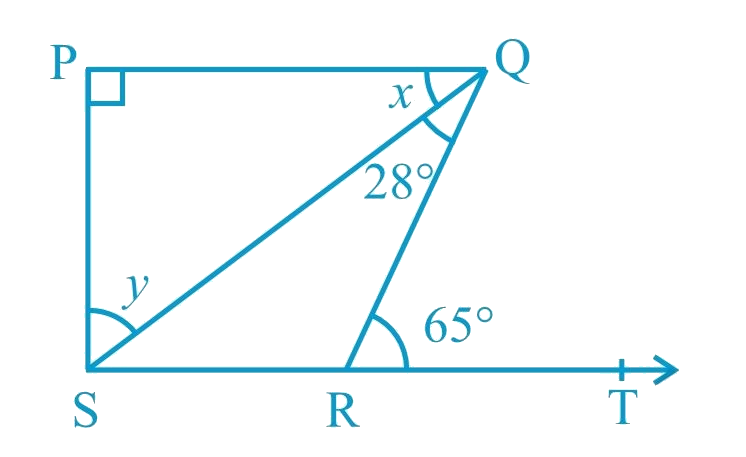### Solution

What is known?

$$PQ ⊥ PS,\, PQ || SR$$,  $$\angle SQR = 28^\circ$$ and $$\angle QRT = 65^\circ$$

What is unknown?

$$x$$ and $$y$$.

Reasoning:

As we know when two parallel lines are cut by a transversal, alternate interior angles formed are equal.

Angle sum property of a triangle:

Sum of the interior angles of a triangle is $$360^\circ$$.

Steps:

Given,

$$PQ ⊥ PS,\, PQ || SR$$,  $$\angle SQR = 28^\circ$$ and $$\angle QRT = 65^\circ$$

\begin{align}\angle PQR &= \angle QRT\\ \begin{array}{I}(\text{Alternate }\end{array} &\begin{array}{I}\text{interior angles}) \end{array}\\\\ \angle PQS + \angle SQR &= \angle QRT \\ \text{(By figure)}\\\\x + 28^\circ &= 65^\circ \\x &= 65^\circ - 28^\circ \\x &= 37^\circ \end{align}

Now, in $$\Delta PQS$$

\begin{align}\angle PQS + \angle PSQ + \angle QPS &=180^\circ\\ ( \text{Angle sum property of a }& \text{triangle}\rm{.})\\ \\37^\circ + y + 90^\circ &= 180^\circ \\y &=180^\circ \!- \!127^\circ \\y &=\, 53^\circ \end{align}

Hence, $$x = 37^\circ$$ and $$y = 53^\circ$$

## Chapter 6 Ex.6.3 Question 6

In Fig. below, the side $$QR$$ of $$\Delta PQR$$ is produced to a point $$S$$. If the bisectors of $$\angle PQR$$ and $$\angle PRS$$ meet at point $$T$$, then prove that \begin{align} \angle QTR = \frac{1}{2}\angle QPR\end{align} .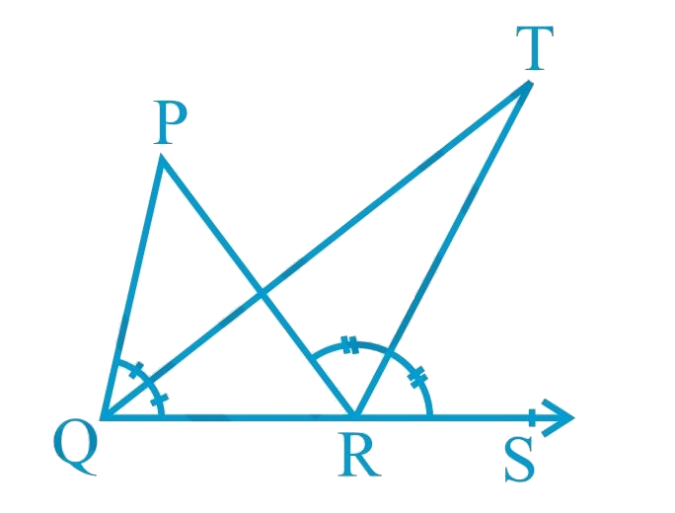### Solution

What is known?

The side $$QR$$ of $$\Delta PQR$$ is produced to a point $$S$$ and the bisectors of $$\angle PQR$$ and $$\angle PRS$$ meet at point $$T$$.

To prove:

\begin{align}\angle QTR = \frac{1}{2}\angle QPR\end{align}

Reasoning:

As we know exterior angle of a triangle:

If a side of a triangle is produced, then the exterior angle so formed is equal to the sum of the two interior opposite angles.

Steps:

Given,

Bisectors of $$\angle PQR$$ and $$\angle PRS$$ meet at point $$T$$.

Hence, $$TR$$ is a bisector of $$\angle PRS$$ and $$TQ$$ is a bisector of $$\angle PQR$$

\begin{align} \angle PRS &= 2\angle TRS\quad\dots\left( {\rm{i}} \right)\\\angle PQR &= 2\angle TQR\quad\dots\left( {{\rm{ii}}} \right)\end{align}

Now, in $$\Delta TQR$$

\begin{align}\angle TRS&= \angle TQR + \angle QTR\\ &\left( {{\text{Exterior angle of triangle}}} \right)\\\\\angle QTR &= \angle TRS-\angle TQR\quad\dots\left( {{\text{iii}}} \right)\end{align}

Similarly, in $$\Delta PQR$$

\begin{align}\angle PRS &= \angle QPR + \angle PQR\qquad\\ &\left( {{\text{Exterior angle of triangle}}} \right)\\\\2\angle TRS &= \angle QPR + 2\angle TQR\\ &\left[ {{\text{From }}\left( {\rm{i}} \right){\rm{ and }}\left( {{\rm{ii}}} \right)} \right]\\\\\angle QPR &= 2\angle TRS-2\angle TQR\\\angle QPR &= 2\left( {\angle TRS-\angle TQR} \right)\\\angle QPR &= 2\angle QTR\qquad\qquad\,\,\,\,\,\qquad\\ &\left[ {{\text{From }}\left( {{\rm{iii}}} \right)} \right]\\\\\angle QTR &= \frac{1}{2}\angle QPR\end{align}

Hence proved.

Related Sections
Related Sections
Instant doubt clearing with Cuemath Advanced Math Program